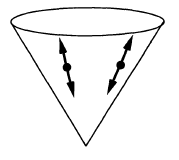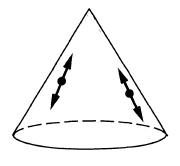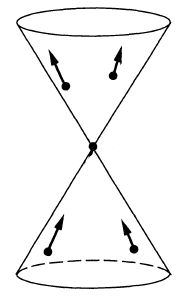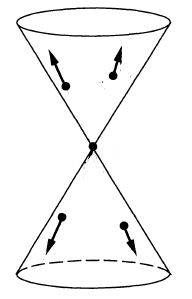# nLab Klein-Gordon equation

Contents

### Context

#### Variational calculus

variational calculus

## Derived differential geometric version

#### Riemannian geometry

Riemannian geometry

# Contents

## Idea

The Klein-Gordon equation is the linear partial differential equation which is the equation of motion of a free scalar field of possibly non-vanishing mass $m$ on some (possibly curved) spacetime (Lorentzian manifold): it is the relativistic wave equation with inhomogeneity the mass $m^2$.

The structure of the Klein-Gordon equation appears also in the equations of motion of richer fields than just scalar fields, where now the underlying field bundle may more generally be some vector bundle. Therefore the fundamental solutions of the Klein-Gordon equation, called the propagators (see below) pervades all of relativistic perturbative quantum field theory.

## Definition

Given a spacetime $(X,g)$ (a pseudo-Riemannian manifold) and a real number $m \in \mathbb{R}_{\geq 0}$, then the Klein-Gordon equation is the differential equation on smooth functions $\phi \colon X \to \mathbb{R}$ given by

$\left( \Box_g - \left( \tfrac{m c}{\hbar} \right)^2 \right) \phi \;=\; 0 \,,$

where $\Box_g$ denotes the wave operator on $(X,g)$ (the analog of the Laplace operator in Lorentzian geometry) and where $\tfrac{m c }{\hbar}$ is for the purpose of pure PDE theory just a real number, while regarded as equipped with physical units it is the inverse Compton wavelength for mass $m$.

This is the equation of motion of the free scalar field on $X$, of mass $m$ and subject to a background field of gravity as encoded in the metric $g$.

## Examples

If $(X,g) = \mathbb{R}^{p,1}$ is Minkowski spacetime equipped with its canonical coordinate functions $x^0 = c t$ and $\{x^i\}_{i = 1}^p$, then the Klein-Gordon equation reads as follows (using Einstein summation convention)

$\left( \eta^{\mu \nu} \frac{\partial}{\partial x^\mu} \frac{\partial}{\partial x^\nu} - \left( \tfrac{m c}{\hbar} \right)^2 \right) \phi \;=\; 0$

hence

$\left( -\tfrac{1}{c^2} \frac{\partial^2}{\partial t^2} + \underoverset{i = 1}{p}{\sum}\frac{\partial}{\partial x^i} \frac{\partial}{\partial x^i} - \left( \tfrac{m c}{\hbar} \right)^2 \right) \phi \;=\; 0$

## Properties

###### Example

Let $\Sigma = \mathbb{R}^{p,1}$ be Minkowski spacetime with Minkowski metric $\eta$ and let $E \coloneqq \Sigma \times \mathbb{R}$ be the trivial line bundle. The canonical volume form $dvol_\Sigma$ induces an isomorphism $\tilde E^\ast \simeq E$.

Consider then the Klein-Gordon operator

$(\Box - m^2) \;\colon\; \Gamma_\Sigma(\Sigma \times \mathbb{R}) \longrightarrow \Gamma_\Sigma(\Sigma \times \mathbb{R}) \otimes \langle dvol_\Sigma\rangle \,.$

This is its own formal adjoint witnessed by the bilinear differential operator given by

$K(\Phi_1, \Phi_2) \;\coloneqq\; \left( \frac{\partial \Phi_1}{\partial x^\mu} \Phi_2 - \Phi_1 \frac{\partial \Phi_2}{\partial x^\mu} \right) \eta^{\mu \nu}\iota_{\partial_\nu} dvol_\Sigma \,.$
###### Proof
\begin{aligned} d K(\Phi_1, \Phi_2) & = d \left( \frac{\partial \Phi_1}{\partial x^\mu} \Phi_2 - \Phi_1 \frac{\partial \Phi_2}{\partial x^\mu} \right) \eta^{\mu \nu}\iota_{\partial_\nu} dvol_\Sigma \\ &= \left( \left( \eta^{\mu \nu}\frac{\partial^2 \Phi_1}{\partial x^\mu \partial x^\nu} \Phi_2 + \eta^{\mu \nu} \frac{\partial \Phi_1}{\partial x^\mu} \frac{\partial \Phi_2}{\partial x^\nu} \right) - \left( \eta^{\mu \nu} \frac{\partial \Phi_1}{\partial x^\nu} \frac{\partial \Phi_2}{\partial x^\mu} + \Phi_1 \eta^{\mu \nu} \frac{\partial^2 \Phi_2}{\partial x^\nu \partial x^\mu} \right) \right) dvol_\Sigma \\ & = \left( \eta^{\mu \nu}\frac{\partial^2 \Phi_1}{\partial x^\mu \partial x^\nu} \Phi_2 - \Phi_1 \eta^{\mu \nu} \frac{\partial^2 \Phi_2}{\partial x^\nu \partial x^\mu} \right) dvol_\Sigma \\ & = \Box(\Phi_1) \Phi_2 - \Phi_1 \Box (\Phi_2) \end{aligned}

### Bicharacteristic flow and propagation of singularities

The bicharacteristic strips of the Klein-Gordon operator are cotangent vectors along lightlike geodesics (this example).

### Fundamental solutions

On a globally hyperbolic spacetime $M$ the Klein-Gordon equation has unique advanced and retarded Green functions, $\Delta_R \in \mathcal{D}'(M\times M)$ and $\Delta_A \in \mathcal{D}'(M\times M)$ respectively.

The advanced and retarded Green functions are uniquely distinguished by their support properties. Namely, $(x,y) \in \operatorname{supp} \Delta_R$ only if $x$ is in the causal future of $y$, while $(x,y) \in \operatorname{supp} \Delta_A$ only if $x$ is in the causal past of $y$.

Their difference $\Delta_S = \Delta_R - \Delta_A$ is a bisolution known as the causal propagator, which is the Peierls bracket which gives the Poisson bracket on the covariant phase space of the free scalar field. This in turn defines the Wick algebra of the free scalar field, which yields the quantization of the free scalar field to a quantum field theory.

Other important Green functions or bisolutions include any (anti-)Feynman propagator ($\Delta_{\bar{F}}$) $\Delta_F$ and Hadamard propagator. Unfortunately, it is not possible to identify them by a simple support condition. On Minkowski space, they are identified by the support of their Fourier transform. On curved spacetimes, there are two possibilities. One specifies the asymptotic expansion $\Delta(x,y)$ in a geodesically convex neighborhood of the diagonal $x=y$ to be of a special Hadamard form. The other specifies constraints on the wavefront set $WF(\Delta)$. The possibilities were proven to be equivalent in Radzikowski 96, which made essential use of the relevant notions of microlocal analysis and of distinguished parametrices introduced in DuistermaatHörmander 72.

According to Radzikowski 96, the constraints on the wavefront sets of important Green functions and bisolutions can be diagrammatically illustrated as follows below. Note that the primed wavefront set of a distribution on $M\times M$ is defined as $WF'(\Delta) = \{ (x,y;p,-q) \in T^*(M\times M) \mid (x,y;p,q) \in WF(\Delta) \}$. The diagrams illustrate tuples $(x,y; p,q) \in T^*(M\times M)$, where the vertex of a cone corresponds to $y$ and the cone illustrates all points $x$ linked to $y$ by null geodesics; the arrows illustrate the allowed directions of $p$, with $p$ and $q$ linked by parallel transport and both tangent to null geodesic linking $x$ and $y$.

propagators (i.e. integral kernels of Green functions)
for the wave operator and Klein-Gordon operator
on a globally hyperbolic spacetime such as Minkowski spacetime:

namesymbolwave front setas vacuum exp. value
of field operators
as a product of
field operators
causal propagator\begin{aligned}\Delta_S & = \Delta_+ - \Delta_- \end{aligned}$\phantom{A}\,\,\,-$\begin{aligned} & i \hbar \, \Delta_S(x,y) = \\ & \left\langle \;\left[\mathbf{\Phi}(x),\mathbf{\Phi}(y)\right]\; \right\rangle \end{aligned}Peierls-Poisson bracket
advanced propagator$\Delta_+$\begin{aligned} & i \hbar \, \Delta_+(x,y) = \\ & \left\{ \array{ \left\langle \; \left[ \mathbf{\Phi}(x),\mathbf{\Phi}(y) \right] \; \right\rangle &\vert& x \geq y \\ 0 &\vert& y \geq x } \right. \end{aligned}future part of
Peierls-Poisson bracket
retarded propagator$\Delta_-$\begin{aligned} & i \hbar \, \Delta_-(x,y) = \\ & \left\{ \array{ \left\langle \; \left[\mathbf{\Phi}(x),\mathbf{\Phi}(y) \right] \; \right\rangle &\vert& y \geq x \\ 0 &\vert& x \geq y } \right. \end{aligned}past part of
Peierls-Poisson bracket
Wightman propagator\begin{aligned} \Delta_H &= \tfrac{i}{2}\left( \Delta_+ - \Delta_-\right) + H\\ & = \tfrac{i}{2}\Delta_S + H \\ & = \Delta_F - i \Delta_- \end{aligned}\begin{aligned} & \hbar \, \Delta_H(x,y) \\ & = \left\langle \; \mathbf{\Phi}(x) \mathbf{\Phi}(y) \; \right\rangle \\ & = \underset{ = 0 }{\underbrace{\left\langle \; : \mathbf{\Phi}(x) \mathbf{\Phi}(y) : \; \right\rangle}} \\ & \phantom{=} + \left\langle \; \left[ \mathbf{\Phi}^{(-)}(x), \mathbf{\Phi}^{(+)}(y) \right] \; \right\rangle \end{aligned}positive frequency of
Peierls-Poisson bracket,
Wick algebra-product,
2-point function
$\phantom{=}$ of vacuum state
$\phantom{=}$ or generally of
$\phantom{=}$ Hadamard state
Feynman propagator\begin{aligned}\Delta_F & = \tfrac{i}{2}\left( \Delta_+ + \Delta_- \right) + H \\ & = i \Delta_D + H \\ & = \Delta_H + i \Delta_- \end{aligned}\begin{aligned} & \hbar \, \Delta_F(x,y) \\ & = \left\langle \; T\left( \; \mathbf{\Phi}(x)\mathbf{\Phi}(y) \;\right) \; \right\rangle \\ & = \left\{ \array{ \left\langle \; \mathbf{\Phi}(x)\mathbf{\Phi}(x) \; \right\rangle &\vert& x \geq y \\ \left\langle \; \mathbf{\Phi}(y) \mathbf{\Phi}(x) \; \right\rangle &\vert& y \geq x } \right.\end{aligned}time-ordered product

These propagators govern the construction of the Wick algebra of quantum observables of the free scalar field on the given globally hyperbolic spacetime, as well as the further deformation quantization to interacting perturbative quantum field theory on curved spacetimes via causal perturbation theory. See at locally covariant perturbative quantum field theory for more on this.

### Relation to Schrödinger equation

Sometimes the Klein-Gordon equation is thought of as a relativistic refinement of the Schrödinger equation (as one passes from the non-relativistic to the relativistic particle). But this requires some care. A priori the Klein-Gordon equation takes as arguments a field on spacetime, whereas the Schrödinger equation takes as argument a wave function on phase space.

The Klein-Gordon equation is named after Oskar Klein and Walter Gordon.

An overview over the KG propagators on Minkowski spacetime is given in

• Mikica Kocic, Invariant Commutation and Propagation Functions Invariant Commutation and Propagation Functions, 2016 (pdf)

The Hadamard propagator for the Klein-Gordon equation on general globally hyperbolic spacetimes was found in

• Marek Radzikowski, Micro-local approach to the Hadamard condition in quantum field theory on curved space-time, Commun. Math. Phys. 179 (1996), 529–553 (Euclid)

The original reference on the relevant notions of microlocal analysis and distinguished parametrices of the Klein-Gordon equation is

Textbook accounts include